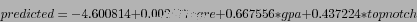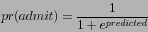DATA MINING
Desktop Survival Guide
by Graham Williams### Tutorial Example

Logistic regression uses the glm command with a binomial (two class) distribution and a logit link function (identified as binomial(logit)).

 > mydata <- read.csv(url("http://www.ats.ucla.edu/stat/r/dae/logit.csv")) > mylogit<- glm(admit ~ gre + gpa + topnotch, data=mydata, family=binomial(link="logit"), na.action=na.pass) > summary(mylogit) Call: glm(formula = admit ~ gre + gpa + topnotch, family = binomial(link = "logit"), data = mydata, na.action = na.pass) Deviance Residuals: Min 1Q Median 3Q Max -1.3905 -0.8836 -0.7137 1.2745 1.9572 Coefficients: Estimate Std. Error z value Pr(>|z|) (Intercept) -4.600814 1.096379 -4.196 2.71e-05 *** gre 0.002477 0.001070 2.314 0.0207 * gpa 0.667556 0.325259 2.052 0.0401 * topnotch 0.437224 0.291853 1.498 0.1341 --- Signif. codes: 0 ‘***’ 0.001 ‘**’ 0.01 ‘*’ 0.05 ‘.’ 0.1 ‘ ’ 1 (Dispersion parameter for binomial family taken to be 1) Null deviance: 499.98 on 399 degrees of freedom Residual deviance: 478.13 on 396 degrees of freedom AIC: 486.13 Number of Fisher Scoring iterations: 4 

As with other summary output of models the first piece of information identifies how the model builder was called.

We can then see the Deviance Residuals. This summary information provides a measure of how well the model fits the data through measuring the deviance between each observation's known target and that predicted by the model. As we would expect, the distribution is spread around zero, and there is not a large spread.

The actual model that is built is then detailed in the following section of the summary. Here, the regression formula, expressed using the scale of the linear predictors for which the model was built (i.e., the predictions are log-odds, or probabilities on the logit scale) is:In R we can see how this works:

 > attach(mydata) > fm <- -4.600814 + 0.002477*gre + 0.667556*gpa + 0.437224*topnotch > head(fm)  -1.24967691 -0.07883943 0.48823400 -0.88603032 -1.35683488 -0.71562600 

To convert this to a predicted probability, we use:> library(e1071) > head(sigmoid(fm))  0.2227561 0.4803003 0.6196903 0.2919297 0.2047552 0.3283569 

Compare this with what the predict function returns:

 > head(predict(mylogit, mydata)) 1 2 3 4 5 6 -1.24974316 -0.07895433 0.48809488 -0.88614116 -1.35692497 -0.71575742 > head(predict(mylogit, mydata, type="response")) 1 2 3 4 5 6 0.2227446 0.4802717 0.6196575 0.2919068 0.2047405 0.3283279 

Todo: Explain minor differences.

The Null model is a model that includes just the intercept.